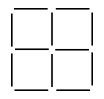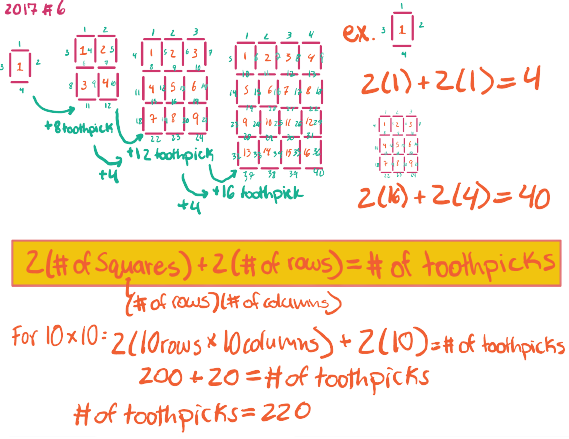# IMAT 2017 Q6 [Matchsticks]

The diagram below shows how 12 matchsticks can be used to create a grid containing 4 squares, arranged in rows of 2.I now intend to use matchsticks to create a grid containing 100 squares, arranged in 10 rows of 10.

How many matchsticks do I need?

A. 220
B. 242
C. 300
D. 200
E. 400

Simple steps to solve word problems:

• Underline key information
• Determine what they are trying to ask, and what you will need to solve it
• Eliminate any non-essential information
• Draw a picture, graph, or equation
• In moments of high stress like exam taking, always work with the paper they give you to avoid careless mistakes.
• Solve.

Approach: this question is attempting to see how well you can formulate an equation that will generalize this pattern. With the information given, you can either spend half the exam time drawing out every single toothpick in a 10x10 square, or you can save your time by drawing out the first few steps and then making an equation you can use to solve the 10x10 problem.

FIrst, start out by drawing squares that follow this rule and find the differences between them.

1 square = 4 toothpicks
4 square = 12 toothpicks
9 square = 24 toothpicks
16 square = 40 toothpicks

Then make a generalized equation that applies to all of the squares. Make sure to test it out before going to solve for the final solution.

2 (# of squares) + 2 (# of rows) = # of toothpicks

Finally, apply this equation to the desired 10x10.

2 (10 rows x 10 columns) + 2 (10 rows) = # of toothpicks
200 + 20 = 220\fcolorbox{red}{grey!30}{Therefore A, \$220\$ is the correct answer.}